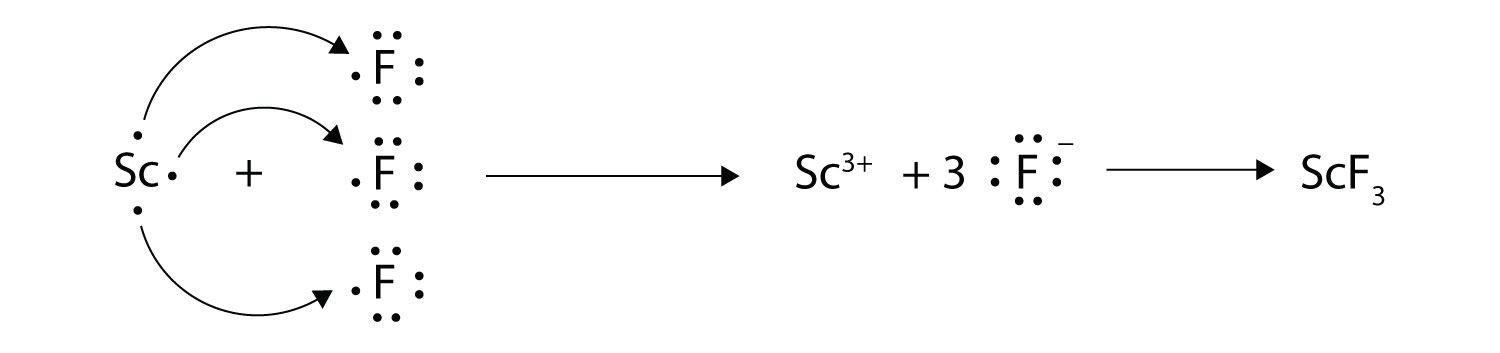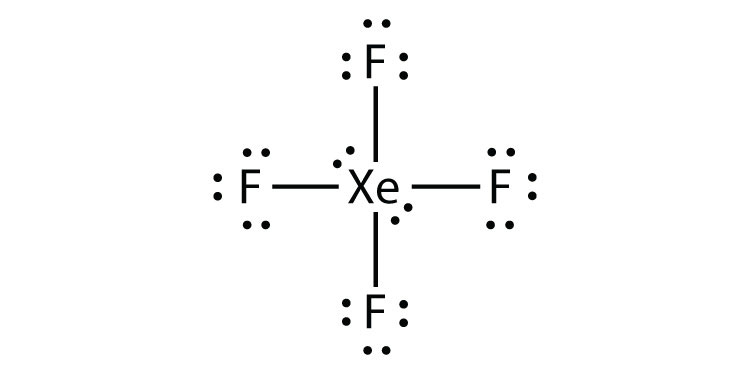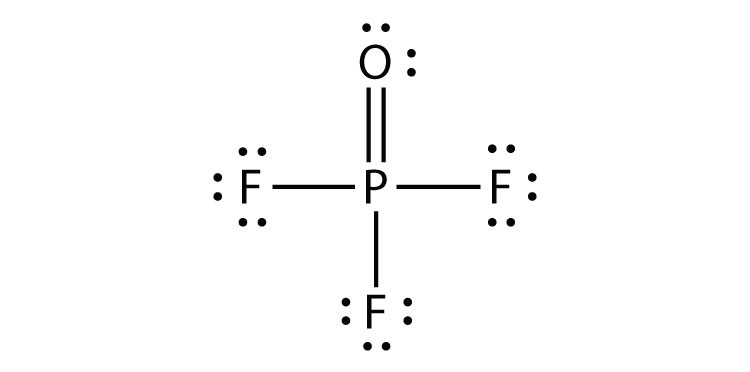# 9.E: Chemical Bonds (Exercises)

•• Contributed by Anonymous
• LibreTexts

## 9.2: Lewis Electron Dot Diagrams

1. Explain why the first two dots in a Lewis electron dot diagram are drawn on the same side of the atomic symbol.
2. Is it necessary for the first dot around an atomic symbol to go on a particular side of the atomic symbol?
3. What column of the periodic table has Lewis electron dot diagrams with two electrons?
4. What column of the periodic table has Lewis electron dot diagrams that have six electrons in them?
5. Draw the Lewis electron dot diagram for each element.
1. strontium
2. silicon
6. Draw the Lewis electron dot diagram for each element.
1. krypton
2. sulfur
7. Draw the Lewis electron dot diagram for each element.
1. titanium
2. phosphorus
8. Draw the Lewis electron dot diagram for each element.
1. bromine
2. gallium
9. Draw the Lewis electron dot diagram for each ion.
1. Mg2+
2. S2−
10. Draw the Lewis electron dot diagram for each ion.
1. In+
2. Br
11. Draw the Lewis electron dot diagram for each ion.
1. Fe2+
2. N3−
12. Draw the Lewis electron dot diagram for each ion.
1. H+
2. H

1. The first two electrons in a valence shell are s electrons, which are paired.
2. the second column of the periodic table
1.2.1.2.1. Mg2+
2.1. Fe2+
2.## 9.3: Electron Transfer - Ionic Bonds

1. Comment on the possible formation of the K2+ ion. Why is its formation unlikely?
2. Comment on the possible formation of the Cl2− ion. Why is its formation unlikely?

3. How many electrons does a Ba atom have to lose to have a complete octet in its valence shell?

4. How many electrons does a Pb atom have to lose to have a complete octet in its valence shell?

5. How many electrons does an Se atom have to gain to have a complete octet in its valence shell?

6. How many electrons does an N atom have to gain to have a complete octet in its valence shell?

7. With arrows, illustrate the transfer of electrons to form potassium chloride from K atoms and Cl atoms.

8. With arrows, illustrate the transfer of electrons to form magnesium sulfide from Mg atoms and S atoms.

9. With arrows, illustrate the transfer of electrons to form scandium fluoride from Sc atoms and F atoms.

10. With arrows, illustrate the transfer of electrons to form rubidium phosphide from Rb atoms and P atoms.

11. Which ionic compound has the higher lattice energy—KI or MgO? Why?

12. Which ionic compound has the higher lattice energy—KI or LiF? Why?

1. Which ionic compound has the higher lattice energy—BaS or MgO? Why?

1. The K2+ ion is unlikely to form because the K+ ion already satisfies the octet rule and is rather stable.
2. two
3. two
4.5.6. MgO because the ions have a higher magnitude charge
7. MgO because the ions are smaller

## 9.4: Covalent Bonds

1. How many electrons will be in the valence shell of H atoms when it makes a covalent bond?
2. How many electrons will be in the valence shell of non-H atoms when they make covalent bonds?
3. What is the Lewis electron dot diagram of I2? Circle the electrons around each atom to verify that each valence shell is filled.
4. What is the Lewis electron dot diagram of H2S? Circle the electrons around each atom to verify that each valence shell is filled.
5. What is the Lewis electron dot diagram of NCl3? Circle the electrons around each atom to verify that each valence shell is filled.
6. What is the Lewis electron dot diagram of SiF4? Circle the electrons around each atom to verify that each valence shell is filled.
7. Draw the Lewis electron dot diagram for each substance.
1. SF2
2. BH4
8. Draw the Lewis electron dot diagram for each substance.
1. PI3
2. OH
9. Draw the Lewis electron dot diagram for each substance.
1. GeH4
2. ClF
10. Draw the Lewis electron dot diagram for each substance.
1. AsF3
2. NH4+
11. Draw the Lewis electron dot diagram for each substance. Double or triple bonds may be needed.
1. SiO2
2. C2H4 (assume two central atoms)
12. Draw the Lewis electron dot diagram for each substance. Double or triple bonds may be needed.
1. CN
2. C2Cl2 (assume two central atoms)
13. Draw the Lewis electron dot diagram for each substance. Double or triple bonds may be needed.
1. CS2
2. NH2CONH2 (assume that the N and C atoms are the central atoms)
14. Draw the Lewis electron dot diagram for each substance. Double or triple bonds may be needed.
1. POCl
2. HCOOH (assume that the C atom and one O atom are the central atoms)

1. two
2.3.1.2.1.2.1.2.1.2.## 9.5: Other Aspects of Covalent Bonds

1. Give an example of a nonpolar covalent bond. How do you know it is nonpolar?
2. Give an example of a polar covalent bond. How do you know it is polar?
3. How do you know which side of a polar bond has the partial negative charge? Identify the negatively charged side of each polar bond.
1. H–Cl
2. H–S
4. How do you know which side of a polar bond has the partial positive charge? Identify the positively charged side of each polar bond.
1. H–Cl
2. N-F
5. Label the bond between the given atoms as nonpolar covalent, slightly polar covalent, definitely polar covalent, or likely ionic.
1. H and C
2. C and F
3. K and F
6. Label the bond between the given atoms as nonpolar covalent, slightly polar covalent, definitely polar covalent, or likely ionic.
1. S and Cl
2. P and O
3. Cs and O
7. Which covalent bond is stronger—a C–C bond or a C–H bond?
8. Which covalent bond is stronger—an O–O double bond or an N–N double bond?
9. Estimate the enthalpy change for this reaction: N2 + 3H2 → 2NH3 .Start by drawing the Lewis electron dot diagrams for each substance.
10. Estimate the enthalpy change for this reaction. Start by drawing the Lewis electron dot diagrams for each substance: HN=NH + 2H2 → 2NH3
11. Estimate the enthalpy change for this reaction. Start by drawing the Lewis electron dot diagrams for each substance: CH4 + 2O2 → CO2 + 2H2O
12. Estimate the enthalpy change for this reaction. Start by drawing the Lewis electron dot diagrams for each substance: 4NH3 + 3O2 → 2N2 + 6H2O

1. H–H; it is nonpolar because the two atoms have the same electronegativities (answers will vary).
1. Cl side
2. S side
1. slightly polar covalent
2. definitely polar covalent
3. likely ionic
2. C–H bond
3. −80 kJ
4. −798 kJ

## 9.6: Violations of the Octet Rule

1. Why can an odd-electron molecule not satisfy the octet rule?
2. Why can an atom in the second row of the periodic table not form expanded valence shell molecules?
3. Draw an acceptable Lewis electron dot diagram for these molecules that violate the octet rule.
1. NO2
2. XeF4
4. Draw an acceptable Lewis electron dot diagram for these molecules that violate the octet rule.
1. BCl3
2. ClO2
5. Draw an acceptable Lewis electron dot diagram for these molecules that violate the octet rule.
1. POF3
2. ClF3
6. Draw an acceptable Lewis electron dot diagram for these molecules that violate the octet rule.
1. SF4
2. BeH2

1. There is no way all electrons can be paired if there are an odd number of them.
1.2.1.2.## 9.7: Molecular Shapes

1. What is the basic premise behind VSEPR?
2. What is the difference between the electron group geometry and the molecular geometry?
3. Identify the electron group geometry and the molecular geometry of each molecule.
1. H2S
2. POCl3
4. Identify the electron group geometry and the molecular geometry of each molecule.
1. CS2
2. H2S
5. Identify the electron group geometry and the molecular geometry of each molecule.
1. HCN
2. CCl4
6. Identify the electron group geometry and the molecular geometry of each molecule.
1. BI3
2. PH3
7. What is the geometry of each species?
1. CN
2. PO43−
8. What is the geometry of each species?
1. PO33−
2. NO3
9. What is the geometry of each species?
1. COF2
2. C2Cl2 (both C atoms are central atoms and are bonded to each other)
10. What is the geometry of each species?
1. CO32−
2. N2H4 (both N atoms are central atoms and are bonded to each other)

1. Electron pairs repel each other.
1. electron group geometry: tetrahedral; molecular geometry: bent
2. electron group geometry: tetrahedral; molecular geometry: tetrahedral
1. electron group geometry: linear; molecular geometry: linear
2. electron group geometry: tetrahedral; molecular geometry: tetrahedral
1. linear
2. tetrahedral
1. trigonal planar
2. linear and linear about each central atom

1. Explain why iron and copper have the same Lewis electron dot diagram when they have different numbers of electrons.
2. Name two ions with the same Lewis electron dot diagram as the Cl ion.

3. Based on the known trends, what ionic compound from the first column of the periodic table and the next-to-last column of the periodic table should have the highest lattice energy?

4. Based on the known trends, what ionic compound from the first column of the periodic table and the next-to-last column of the periodic table should have the lowest lattice energy?

5. P2 is not a stable form of phosphorus, but if it were, what would be its likely Lewis electron dot diagram?

6. Se2 is not a stable form of selenium, but if it were, what would be its likely Lewis electron dot diagram?

7. What are the Lewis electron dot diagrams of SO2, SO3, and SO42−?

8. What are the Lewis electron dot diagrams of PO33− and PO43−?

9. Which bond do you expect to be more polar—an O–H bond or an N–H bond?

10. Which bond do you expect to be more polar—an O–F bond or an S–O bond?

11. Use bond energies to estimate the energy change of this reaction.

C3H8 + 5O2 → 3CO2 + 4H2O
12. Use bond energies to estimate the energy change of this reaction.

N2H4 + O2 → N2 + 2H2O
13. Ethylene (C2H4) has two central atoms. Determine the geometry around each central atom and the shape of the overall molecule.

14. Hydrogen peroxide (H2O2) has two central atoms. Determine the geometry around each central atom and the shape of the overall molecule.

1. Iron has d electrons that typically are not shown on Lewis electron dot diagrams.
2. LiF

3. It would be like N2:4.5. an O–H bond

6. −2,000 kJ

7. trigonal planar about both central C atoms